HOME > FAQ > Motorized Stage(FAQ) > Terms / definition (accuracy, unit, etc.)

### Terms / definition (accuracy, unit, etc.)

Speed setting defined by "pps" (pulse per second)
I am trying to connect KXL06150-N2-FA to DS102NR. I want to set the maximum speed at 25 mm/sec.
I read the instruction manual, but the speed setting is written in pps and I do not understand it well.
How should I calculate?

The answer is based on the assumption that the controller division number setting is FULL step (*).

pps means pulse / sec (read as pulse per sec).
The speed unit needs to be converted from [mm / sec] to [pulse / sec].

The resolution of the KXL06150-N2-FA (catalog value) is “4 μm (FULL)”.
In other words, it moves 4 μm per pulse.

1 [pulse] = 4 [μm]

Convert the velocity to the resolution unit [μm].

(Speed) = 25 [mm / sec] = 25,000 [μm / sec]

Calculate the number of pulses required to move 25,000 μm .

Number of pulses = 25,000 [μm] ÷ 4 [μm / pulse] = 6,250 [pulse]

Therefore, the speed of 25 mm / sec is equivalent to " 6,250 pulse / sec".

* What is FULL step?
Basic step angle determined by the motor structure, which is called FULL step.
For a 5-phase stepping motor, this is generally 0.72 degree.
Some motors have a basic step angle of 0.36 degree, so when calculating the stage resolution from
the motor resolution, it is necessary to check the specifications of the motor used.

For FULL step, there is a technology to subdivide the moving angle per pulse by excitation method
and current control.
These are called HALF step and MICRO step, and HALF is half of the basic step angle
(0.36 deg per pulse when the basic step angle is 0.72 deg),
and micro step is 1/250 of the basic step angle (basic step angle) when it is 0.72 deg, it can be divided up
to 0.00288 deg per pulse).
This makes it possible to reduce the resolution of the stage by reducing the resolution of the motor.

The actual stage movement involves sliding resistance and elastic deformation.
Therefore, even if the resolution of the motor is too small, the operation on the stage side does not
necessarily follow.
In general, 1/8 to 1/20 division is the limit of stage tracking.

Meaning of CW direction, CCW direction
There are CW direction and CCW direction according to the movement direction of the motorized stage.
What does each mean?

The direction of rotation of the motor represents the direction of movement of the stage.
* Direction of rotation of motor = Direction of rotation of motor shaft viewed from the stage table side
(opposite to the knob of motor)

◆ CW (= ClockWise)
The direction in which the table (upper surface of the stage) moves to the opposite side of the motor.

◆ CCW (CounterClockWise)
The direction in which the table (upper surface of the stage) moves to the motor side.

The linear motion stage (XY stage) and goniometer stage are as described above.
In the case of the horizontal Z-axis stage and rotary stage, the moving direction of the stage top table
is as follows.

CW direction: Direction in which the table (upper surface of the stage) goes up
CCW direction: Direction in which the table (upper surface of the stage) goes down

CW direction: Direction in which the table (upper surface of the stage) moves clockwise
CCW direction: Direction in which the table (upper surface of the stage) moves counterclockwise

Start-up speed, Acceleration and deceleration rate, Driving speed
What are the start-up speed (launch speed), acceleration / deceleration rate, driving speed, MAX speed?

・ Start-up speed (Launch speed): This is the speed setting when the stage starts.
・ Acceleration / deceleration rate: The acceleration time that reaches the driving speed from the
start-up speed, and the deceleration time until the stage stops from the driving speed.
・ Driving speed: Speed to drive steadily.
・ MAX speed: The maximum driving speed by setup, which can be different for each stage.

Resolution (motorized stage)
What is the resolution?

The amount by which the stage moves when a single pulse signal is sent.

Full step, Half step, micro-step
What do you mean by the resolution "FULL, HALF, microstep"?

This is the motor's electrical resolution for one pulse.
FULL: Full-step HALF: Half-step MS: Micro-step The resolution of the stage changes depending
on the number of divisions.

◆ HALF (half step)
When half step is set, the movement amount per pulse is half compared to the case of full step.

◆ MS (Micro step)
We can do setting more detailed than Half step.
The division number setting is 16 patterns. (1,2,2.5,4,5,8,10,20,25,40,50,80,100,125,200,250)
* However, the actual following capability depends on the product. It is a standard of about 1/20
in the linear motion stage.
(Not covered by warranty)

Pitching, yawing, rolling
What does "pitching, yawing, rolling" mean?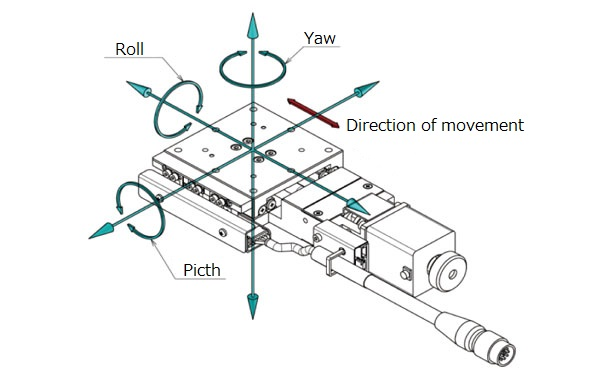◆ Pitching: Rotating movement around the axis perpendicular (left and right) to the traveling direction
(front and back)
→ Inclination of up and down
◆ Yawing: Rotating movement around the axis perpendicular (up and down) to the traveling direction
(front and back)
→ Inclination of left and right
◆ Rolling: Rotating movement around the axis of travelling direction (forward and backward)

Straightness, pitching, yawing
Please tell me the difference between straightness, pitching and yawing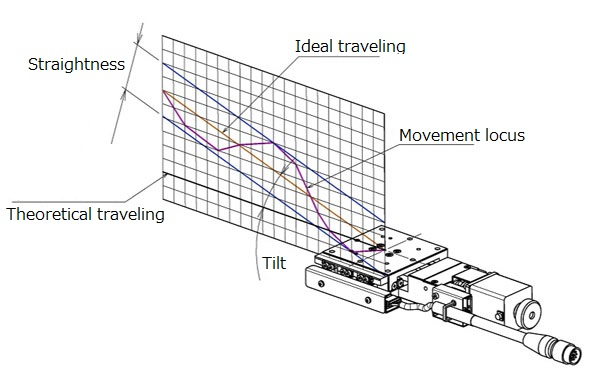◆ Straightness [unit: μm]
・ Maximum displacement amount (distance) in the horizontal and vertical directions with respect
to the ideal movement axis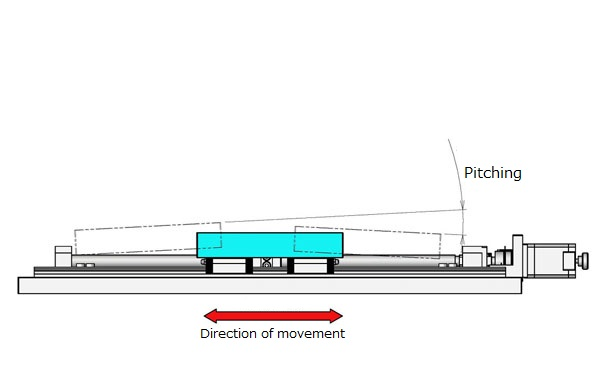◆ Pitching [unit: ″]
・ Maximum displacement angle in the vertical swing direction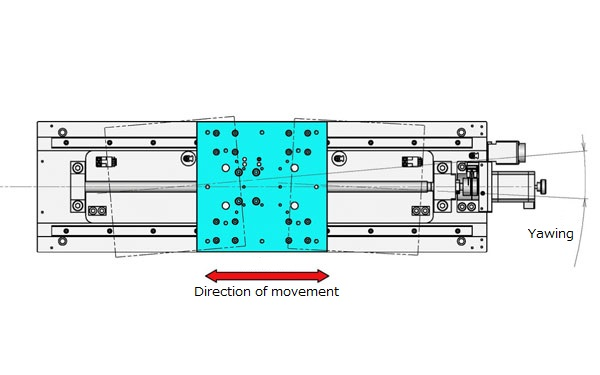◆ Yawing [unit: ″]
・ Maximum displacement angle in the horizontal swing direction

[Rotary stage] Difference between eccentricity and runout
Please tell me the difference between eccentricity and runout of rotary stage

◆ Eccentricity [unit: μm]]
・ Displacement of center axis of rotation in horizontal direction

◆ Runout [unit: μm]]
・ Maximum difference of displacement with respect to the reference plane when making one rotation
with outer circumference as measurement point

Difference between parallelism and motion parallelism
Please tell me the difference between parallelism and motion parallelism

◆ Parallelism [unit: μm]
・ Fix the stage on the reference plane.
・ A numerical value indicating how parallel the stage carriage and the reference plane are.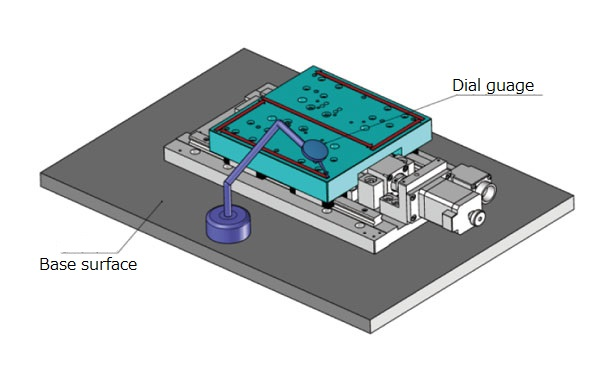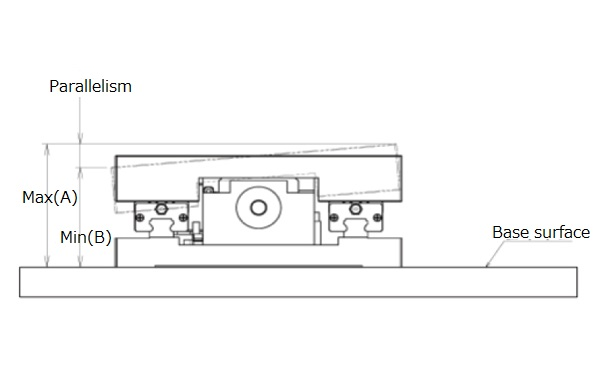◆ Motion parallelism [unit: μm]
・ Fix the stage on the reference plane.
・ Place equipment for measuring the height with the reference plane on the stage carriage.
・ Maximum difference of measured values ​​by moving the stage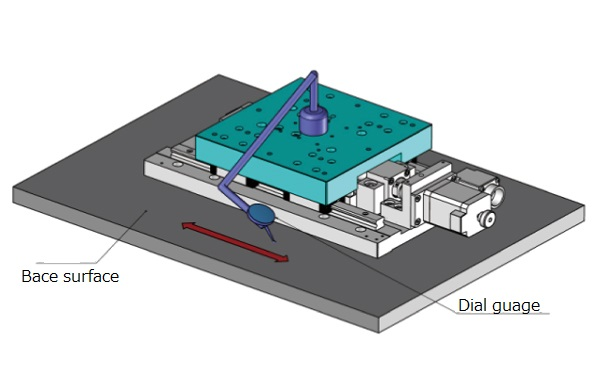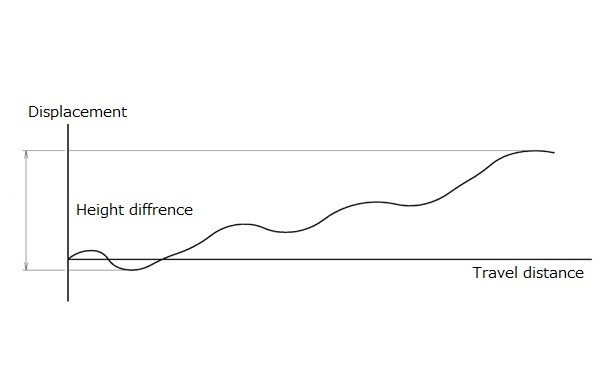Uni-directional positioning accuracy, Repeatability positioning accuracy, Lost motion
Please tell me the difference among uni-directional positioning accuracy, repeatability positioning accuracy and lost motion

◆ Uni-directional positioning accuracy [unit: μm]
A value representing "How much Point B has a possibility of positional deviation when X is
moved from Point A to B?".

(Definition)
Send one-tenth of the total stroke, measure 10 points, and measure the maximum difference between
measured values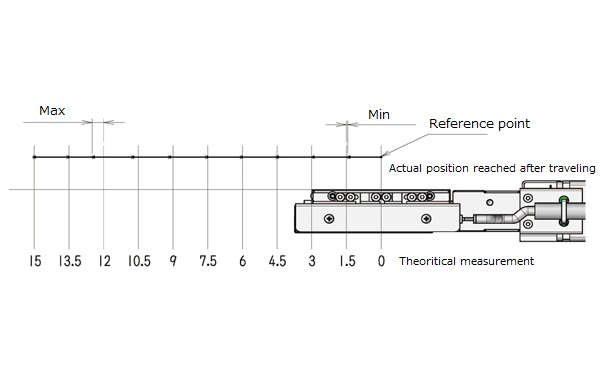◆ Repeatability positioning accuracy [unit: ± μm]
A value representing "When you want to go back and forth the same place, what level of position
shift will occur?"

(Definition)
Repeat the positioning seven times from the same direction for any one point to measure the deviation from the actual stop position.
Calcurate a half of the maximum difference of the measured deviation.
Biggest value among the three points of the center and both ends of the stroke.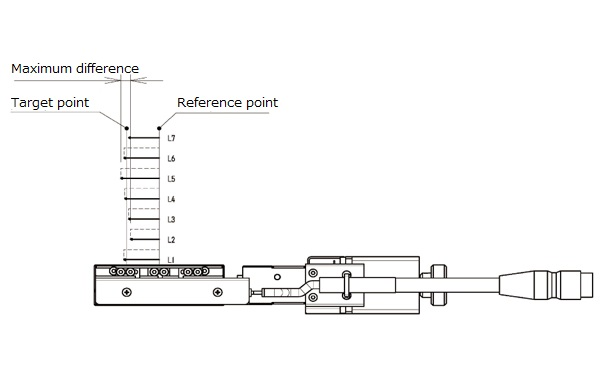◆ Lost motion [unit: μm]
A value representing "What range of deviation will occur when trying to stop at the same place on
both back and forth directions?"

(Definition)
Make positioning toward arbitrary point (point A) from point B.
The actual stop position at this time is the point C. ( * Positioning from the Positive direction )

Move in the opposite direction of point B. --- Point D

····· Make positioning from point D to point A.
The actual stop position at this point is the point E ( * Positioning from the Negative direction )

Repeat this measurement seven times each in the positive and negative directions.
Calcurate the difference between “average of seven measurements at point C” and “average of
seven measurements at point E”.

The largest value when this is done at the 3 points (center of the stroke and both ends).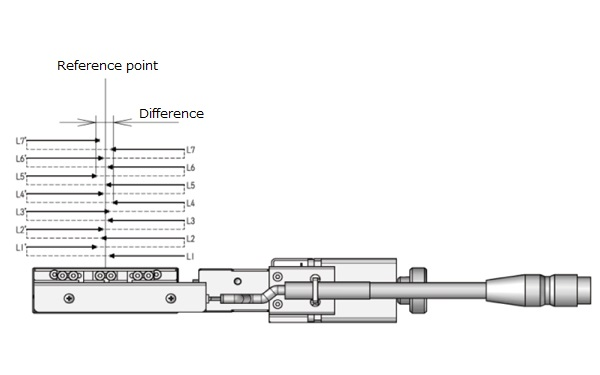Moment rigidity
What is the moment rigidity?

◆ Moment rigidity [Unit: "/ N · cm]
・ The displacement angle per 1 N · cm when moment load is applied toward the stage carriage
in the direction of the respective pitch, yaw & roll
* Based on the distance from the center of the stage carriage (upper surface)
* The moment rigidity value in the catalog is described as a representative actual measurement value.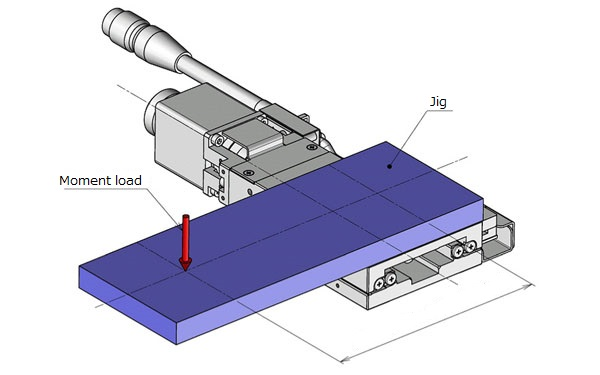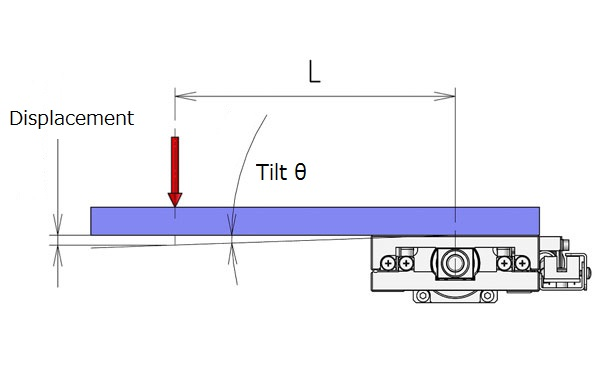Resolution of servo motor option
We are considering the servo motor option of the KXL06 series (1 mm lead type).
What is the minimum resolution of this product?

The servo motor option (option code UA) uses Mitsubishi Electric AC servomotor (model: HG-KR053),
which is used a 22-bit encoder type and can be divided into 4194304 by one motor rotation (360 °).
*4194304 P/R

The stage moves 1 mm (1000 μm) with one rotation.
Therefore, “1000 μm / 4194304 ≒ 0.0002384 μm” is the theoretical minimum resolution.
* It is different from actual following capability. It does not guarantee movement with the above resolution.

What is reverse type (R type)
What kind of direction is the difference of reverse type (R type)?

It is the direction which reflected the motorized stage in the mirror.
The cable connector and limit sensor position will be on the opposite side.

Acceleration and deceleration
How should we calculate acceleration?
Please also tell me the relationship with acceleration / deceleration time.

The acceleration is the rate of change of speed per unit time,
and is expressed as “m / sec^2 (meters per secsec),“ etc.

If it comes to 10 mm / sec in 100 msec (= 0.1 seconds), the acceleration in this case is
10 mm / sec ÷ 100 msec (= 0.1 seconds) = 100 mm / sec^2 (0.1 m / sec^2)
* When the start pulse is 0 (zero)